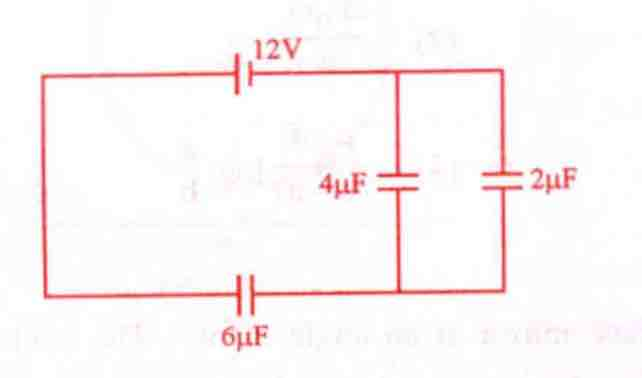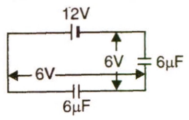# Q. The charge deposited on 4 $\mu$F capacitor in the circuit isKCET KCET 2009Electrostatic Potential And Capacitance

Solution:

## $6 \mu$ F and 6 $\mu$ F are in series $\therefore$ Voltage ascross 4 $\mu$F = 6 V $\therefore$ Q $= 6\times 4 \times 10^{-6}$ $= 24 \times 10^{-6}$ C